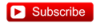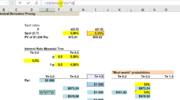#### Nicole Seaman

##### Director of FRM Operations
Staff member
Subscriber
One of the harder ideas in fixed income is risk-neutral probabilities. In this video, I'd like to specifically illustrate, and define, what we mean by risk-neutral probabilities. I will do this in three steps. The first one is just a simple example of a coin toss, where my objective is to illustrate what we mean by risk-neutral probabilities. These are the probabilities that equate the expected discounted value to the market price of the instrument. Then, having defined the concept, in the second sheet I've replicated Bruce Tuckman's example in Chapter 7 where he retrieves the risk-neutral probabilities that are implied by a 1-year zero-coupon bond. We'll take those risk-neutral probabilities and go to the third sheet and use them to price or value an option on that same bond so that's a contingent claim. Then we'll see why there is some magic to these risk-neutral probabilities.#### vinebills

##### New Member
Can sombody help me understand this? I get the fact that we value it in the risk neutral world where we are indifferent about risk. why?
Is it due to the fact that if one were to value it in the risk world there would be arbitrage?. didnt quite get this.

#### prianthar

##### New Member
Subscriber
Hi David,
In the example, if S(1) is 5.15% so a 1yr spot rate, why do we divide it by 2 if its an annual rate to get 950.42? Can see its the same in the text book but its not intuitive, to me at least. So hoping you could elabourate please? Unless, we are saying that it is 5.15 per period and a period is half a year...but then we divide the 5.50 and 4.50 by 2 so all rates seem to be annual so that doesn't work out for me.#### David Harper CFA FRM

##### David Harper CFA FRM
Staff member
Hi @prianthar Rates should (almost) always be expressed in per annum terms; e.g. above the S(0.5) = 5.00% and S(1.0) = 5.15% are inputs and therefore they are per annum (aka, annualized). To discount discretely depends on the compound frequency and where k = the number of periods per year, the general form is:

PV = FV/(1 + r/k)^(T*k); in the above case where the frequency is semi-annual such that k = 2 period per year:
PV = $1,000/(1 + 5.15%/2)^(1*2) =$950.43

Just for example, other variations
PV = $1,000/(1 + 5.15%/1)^(1*1) =$951.022; i.e., discounted one (T = 1) year at 5.51% per annum with annual (k = 1) compound frequency
PV = $1,000/(1 + 5.15%/2)^(3*2) =$858.521; i.e., discounted three (T = 2) years at 5.51% per annum with semi-annual (k = 2) compound frequency
PV = $1,000/(1 + 5.15%/4)^(5*4) =$774.253; i.e., discounted five (T = 5) years at 5.51% per annum with quarterly (k = 4) compound frequency
PV = $1,000/(1 + 5.15%/1)^(5*1) =$777.953; i.e., discounted five (T = 5) years at 5.51% per annum with annual (k = 1) compound frequency